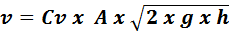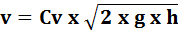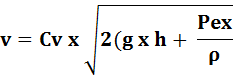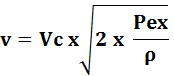## Mechanics of liquids (MEC215)

Hydrotronics can be use in some automation and robotic applications complementing electronic and electronics resources. In this article, from my book Mechatronics and Robotics (out os print), we describe some formulas and applications of liquids devices in mechatronics.

When using hydraulics or hydronics in mechatronics projects the designer must know how the devices work and this implies to know the fundamentals concepts and laws of the mechanics of liquids. In the next lines we will give to the reader some formulas, tables, applications and basic concepts involving hydrotronics (*).

(*) We can define "hydrotronics" the science derived of the application at the same time of electronics and hydraulics.

## Pressure

a) Pressure is the amount of force for unit of area..

Formula

## P = F/S

Where:

P is the pressure

F is the force

S is the area where the force is applied

b) Pressure of a column of liquid.

Formula

## P = ρ x g x h

Where:

P is the pressure

ρ is the density of the liquid

g is the acceleration of gravity (9.8 m/s2)

h is the height of the column

c) Pressure in determinated depth of water:

Formula

## P = h x D

Where:

P is the pressure

h is the depth of the considered point

D is the density of the water (or other liquid)

Table

Density of Water in Different Temperatures

 T (°C) D (g/mL) 0 0.999 87 1 0.999 93 2 0.999 97 3 0.999 99 4 1.000 00 5 0.999 99 6 0.999 97 7 0.999 93 8 0.999 88 9 0.999 81 10 0,999 73 11 0.999 63 12 0.999 52 13 0.999 40 14 0.009 27 15 0.999 13 16 0.998 97 17 0.998 80 18 0.998 62 19 0.998 43 20 0.998 23 21 0.997 02 22 0.997 80 23 0.997 57 24 0.997 32 25 0.997 07 26 0.996 81 27 0.996 52 28 0.996 22 29 0.995 92 30 0.995 61

Table

Density of Some Liquids

(at 20o C)

 Liquid Density (g/cm3) Acetic acid 1.049 Acetone 0.791 Aniline 1.02 Benzene 0.879 Benzine 0.68 - 0.72 Chloroform 1.489 Crude Oil 0.76 - 0.86 Ethyl Alcohol 0.79 Formic acid 1.22 Glycerine 1.26 Heavy Water 1.108 6 Heptane 0.684 Hexane 0.660 Machine oil 0.8 - 0.9 Mercury 13.55 Methyl Alcohol 0.792 Milk (average) 1.03 Nitric acid 1.51 Nitroglycerine 1.6 Sea water 1.01 - 1.03 Water 0.998 23

## Archimedes' Principle

A body, immersed in a liquid is buoyed up by a force equal to the weight of the liquid displaced.## Communicating vessels

The heigths of columns in communicating vessels are inversely proportional to their densities.Formula

## h1/h2 = d2/d1

Where:

h1 and h2 are the heigths of columns

d1, d2 are the denseities of the liquids

## Flow of Liquids from Containers

The speed of the liquid in the outlet depends on several factor as shape, liquid density, position of the outlet in the container, etc. Figure 3 shows some cases with the associated formulas bellow.

a) Base Aperture

Formulab) Small Lateral Aperture

Formulac) Excess Pressure on Surface of Liquid

Formulad) Excess of pressure applied to na outlet point

FormulaWhere:

v is the velocity in the outlet

Cv is the velocity coefficient (0.97 for water)

g is the gravity acceleration (9.8 m/s2)

h is the heith of the container filled with water

Pex /? is pressure in excess by area unit